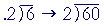S k i l l
i n
A R I T H M E T I C

Lesson 13

# DIVIDING DECIMALSIn Lesson 12, Question 3, we saw how to proceed when the Dividend is a decimal. There remains only the case in which the Divisor is a decimal. But the Divisor must be a whole number. Making it a whole number depends on this fact:

If we multiply the dividend and divisor by the same number,
the quotient will not change.We saw that in Lesson 11 where we used the division bar, which is now the most useful way to indicate division. (See Problems 30 - 31 of that Lesson.) For the sake of those students still required to learn division with the traditional division box:

 When the divisor is a decimal, what must we do?1) Multiply the divisor by 10, or 100, or 1000, etc., according to the number of decimal digits, so that it becomes a whole number. 2) Multiply the dividend by the same power of 10.

14 ÷ .02 = 1400 ÷ 2 = 700.Multiply the divisor by 100 so that it becomes the whole number 3.  Compensate by multiplying 12 by 100 also; add on two 0's.  (Lesson 4, Questions 1 and 2.)Make .008 into the whole number 8 by multiplying it by 1000.  Multiply the dividend also by 1000 ---- move the decimal point three places right.  (Lesson 4, Question 2.)

Notice that the dividend becomes a whole number.  There is no decimal point.  Therefore there will be no decimal point in the quotient:Multply both numbers by 10.  The decimal point then goes directly above.

Example 4.   8.3 ÷ 0.04

Solution.   Here is how to do this using the division bar. It goes directly to the meaning of division rather than simply skill in a method.

 8.3.04 = 830  4 = 800 + 28 + 2          4 , on decomposing 800   into as many multiples  of 4 as possible,
 = 200 + 7 + 24
 = 200 + 7 + 12
 = 207.5, according to Lesson 24.

These examples illustrate that, even though we are dividing decimals, we can really divide only whole numbers, and then correctly place the decimal point.  The same is true for multiplying decimals, adding them and subtracting them.

At this point, please "turn" the page and do some Problems.

or

Continue on to the next Lesson.

1st Lesson on Division